2020 NBA Sixth Man of the Year Odds
+300
3 to 1Jordan Clarkson
17.7% implied probability

+800
8 to 1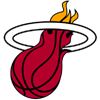Goran Dragic
7.9% implied probability

+1000
10 to 1Terrence Ross
6.4% implied probability

+1200
12 to 1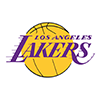Montrezl Harrell
5.4% implied probability

+1200
12 to 1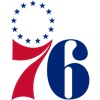Shake Milton
5.4% implied probability

+1400
14 to 1Chris Boucher
4.7% implied probability

+1600
16 to 1Lou Williams
4.2% implied probability

+2000
20 to 1Caris LeVert
3.4% implied probability

+3300
33 to 1Eric Gordon
2.1% implied probability

+3300
33 to 1Austin Rivers
2.1% implied probability

+3300
33 to 1Derrick Rose
2.1% implied probability

+3300
33 to 1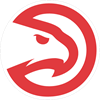Bogdan Bogdanovic
2.1% implied probability

+3300
33 to 1Bobby Portis
2.1% implied probability

+3300
33 to 1Gary Trent
2.1% implied probability

+3300
33 to 1Danilo Gallinari
2.1% implied probability

+5000
50 to 1Trey Burke
1.4% implied probability

+5000
50 to 1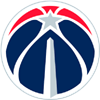Davis Bertans
1.4% implied probability

+5000
50 to 1Jarrett Allen
1.4% implied probability

+5000
50 to 1Alec Burks
1.4% implied probability

+5000
50 to 1Joe Ingles
1.4% implied probability

+5000
50 to 1Norman Powell
1.4% implied probability

+8000
80 to 1Luke Kennard
0.9% implied probability

+8000
80 to 1Aaron Holiday
0.9% implied probability

+8000
80 to 1Brandon Clarke
0.9% implied probability

+8000
80 to 1Kevin Huerter
0.9% implied probability

+8000
80 to 1Carmelo Anthony
0.9% implied probability

+8000
80 to 1DeMarcus Cousins
0.9% implied probability

+10000
100 to 1Rodney Hood
0.7% implied probability

+10000
100 to 1Derrick Favors
0.7% implied probability

+10000
100 to 1Larry Nance
0.7% implied probability

+10000
100 to 1Rajon Rondo
0.7% implied probability

+10000
100 to 1Cameron Johnson
0.7% implied probability

+10000
100 to 1Eric Paschall
0.7% implied probability

+10000
100 to 1JJ Redick
0.7% implied probability

+10000
100 to 1Grant Williams
0.7% implied probability

+10000
100 to 1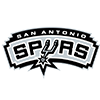Keldon Johnson
0.7% implied probability

+10000
100 to 1Jeff Teague
0.7% implied probability

+10000
100 to 1De'Anthony Melton
0.7% implied probability

+10000
100 to 1Enes Kanter
0.7% implied probability

+10000
100 to 1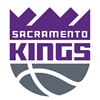Cory Joseph
0.7% implied probability

+10000
100 to 1Markieff Morris
0.7% implied probability

+10000
100 to 1Josh Hart
0.7% implied probability

+10000
100 to 1Monte Morris
0.7% implied probability

+10000
100 to 1Kyle Kuzma
0.7% implied probability

+15000
150 to 1Landry Shamet
0.5% implied probability

+15000
150 to 1Obi Toppin
0.5% implied probability

+15000
150 to 1JaMychal Green
0.5% implied probability

+15000
150 to 1Dwight Howard
0.5% implied probability

+15000
150 to 1Kevin Porter
0.5% implied probability

+20000
200 to 1Bol Bol
0.4% implied probability

+20000
200 to 1Matisse Thybulle
0.4% implied probability

+20000
200 to 1Jabari Parker
0.4% implied probability

+20000
200 to 1Torrey Craig
0.4% implied probability

+20000
200 to 1Brad Wanamaker
0.4% implied probability

+20000
200 to 1D.J. Augustin
0.4% implied probability

+20000
200 to 1Andre Iguodala
0.4% implied probability